Describing motion along a line

Start this free course now. Just create an account and sign in. Enrol and complete the course for a free statement of participation or digital badge if available.

Free course

# 4.4 Velocity and acceleration as derivatives

Recalling that the instantaneous velocity of a particle at time t is given by the gradient of its position-time graph at that time, we can now use the terminology of functions and derivatives to say that the velocity of the particle is given by the derivative of its position function. In terms of symbols: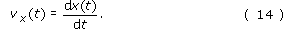Similarly, we can say that the instantaneous acceleration of a particle is given by the derivative of the velocity function: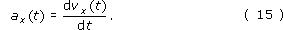What's more we can use derivatives to simplify problems, as Example 1 shows.

## Example 1

The position x of a particle at time t is given by the function x﻿(﻿t﻿) = kt﻿2 where k = −5 m s −2. Find (a) the velocity as a function of time; (b) the velocity at time t = 3 s.

(a) The velocity vx is the derivative with respect to time of the position function x﻿(t) which is of the form At﻿n, with A = k and n = 2. It therefore follows from the third of the standard results in Table 6 that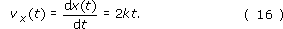This is the required answer. No measuring of the gradients of tangents to curves is involved!

(b) Remembering that k is given as −5 m s−2, the velocity at time t = 3 s is now easily obtained from Equation 16, as follows

vx(3 s) = 2kt = 2 × (−5 m s−2) × (3 s) = −30 m s−1

Note that we have multiplied m s﻿−2 by s to give m s﻿−﻿1, i.e. the units have been treated just like algebraic quantities.

## Question 17

If the velocity v﻿x﻿(﻿t﻿) of a particle is given by v﻿x﻿(﻿t﻿) = kt﻿2, where k = 4 m s﻿−3, find a general expression for the acceleration ax(t). What is the value of a﻿x﻿(﻿3 s﻿)?

The expression for the acceleration is given by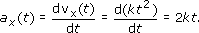using Table 6. Therefore ax(3 s) = (2 × 4 × 3) m s−2 = 24 m s−2.

Although it would be quite wrong to think of dx/dt as a ratio of the quantities dx and dt, it is useful to regard dx/dt as consisting of an entity d/dt that acts on the function x(t). The entity d/dt is a mathematical instruction to differentiate the function that follows, x﻿(﻿t﻿) in this case. It is an example of what a mathematician would call an operator. Adopting this view, we can say that if, for example, x﻿(﻿t﻿) = kt﻿2 + ct, then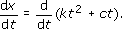Using the rule for differentiating the sum of two functions, from Table 6, we may write this as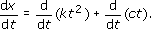Using the third result in Table 6 we may work out both the derivatives on the right-hand side to obtain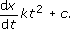Regarding d/dt as an operator also suggests another way of writing the acceleration. We already know that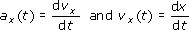so it seems sensible to write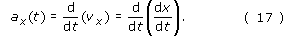This emphasises that the acceleration of a particle is the rate of change of the rate of change of the position, or if you prefer, the derivative of the derivative of the position function. Either of these formulations is a bit of a mouthful, so it is more conventional to refer to the acceleration as the second derivative of x(t) and to represent it symbolically by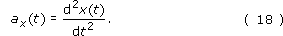Once again, it would be quite wrong to think of this as some kind of ratio of d﻿2﻿x and d﻿t﻿2; it simply indicates that a particle's position function, x﻿(﻿t﻿), must be differentiated twice in order to find the particle's acceleration.

Note: If you are not already familiar with derivatives you should pay particular attention to the positioning of the superscripts in the second derivative symbol. Newcomers to differentiation often make the mistake of writing dx﻿2﻿/﻿d﻿t﻿2 when they mean d﻿2﻿x﻿/﻿d﻿t﻿2. Remembering that it is the operator d/dt that is to be squared rather than the function x﻿(﻿t﻿) may help you to avoid this error.

## Example 2

Suppose, as in Example 1, the position of a particle is given by x﻿(﻿t﻿) = kt﻿2 where k is a constant. Find a﻿x﻿(﻿t﻿).

We use Equation 14 and the third result in Table 6 with A = k, y = t and n = 2, to obtainIt then follows from Equation 18, that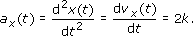where we have again used the third result in Table 6 but with A = 2k and n = 1.

## Question 18

Suppose that the vertical position x of a test vehicle falling down a drop-shaft is given by the quadratic function

x(t) = k0 + k1t + k2t2

where k0, k1 and k2 are constants. Work out an expression for the vehicle's acceleration a﻿x﻿(﻿t﻿) in terms of k﻿0, k﻿1 and k﻿2, taking care to indicate each step in your working.

Using Equations 14 and 15 and Table 6, we find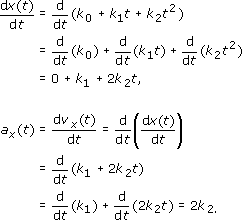S207_2